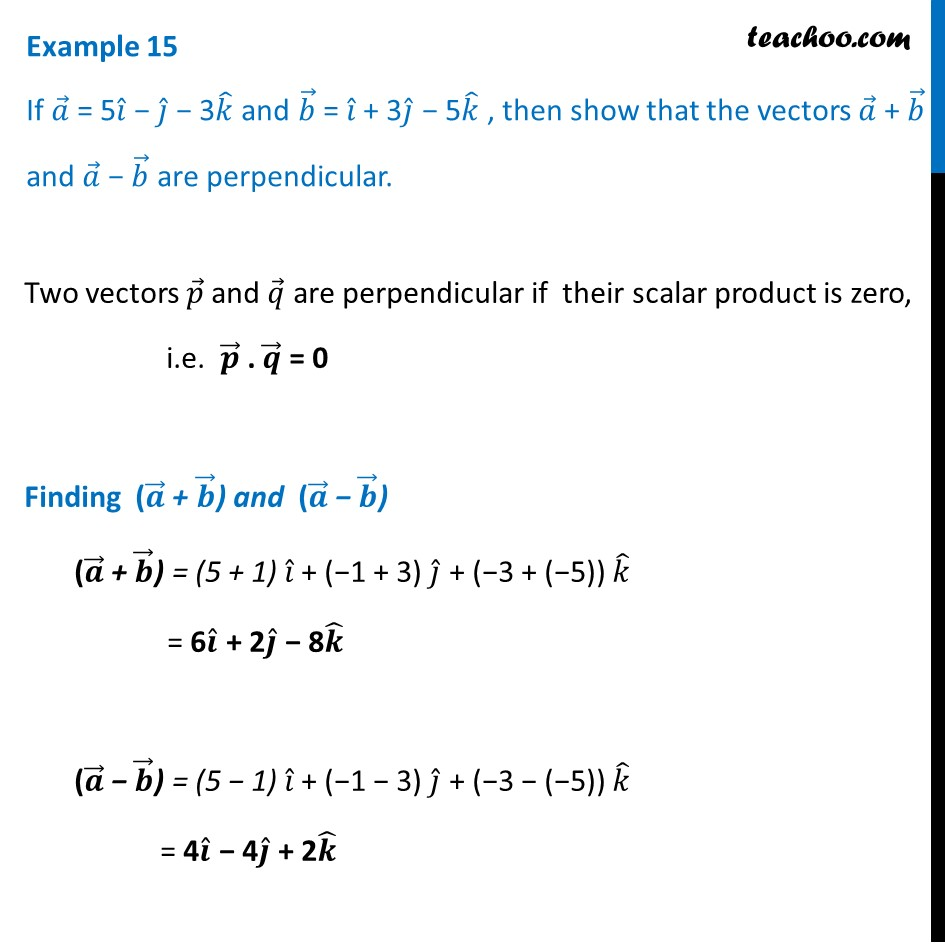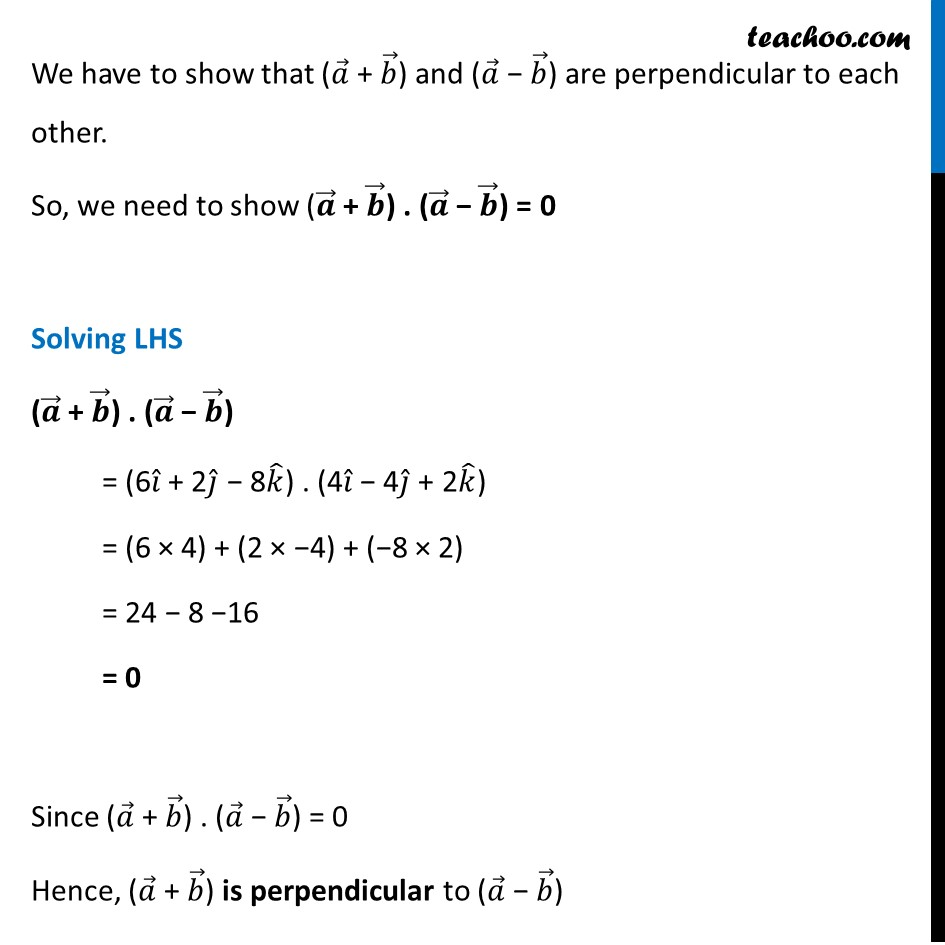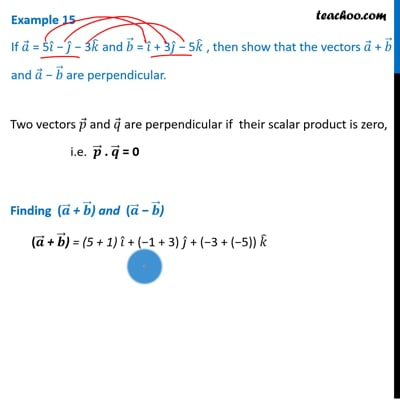Examples

Chapter 10 Class 12 Vector Algebra
Serial order wiseThis video is only available for Teachoo black users

Learn in your speed, with individual attention - Teachoo Maths 1-on-1 Class

### Transcript

Example 15 If 𝑎 ⃗ = 5𝑖 ̂ − 𝑗 ̂ − 3𝑘 ̂ and 𝑏 ⃗ = 𝑖 ̂ + 3𝑗 ̂ − 5𝑘 ̂ , then show that the vectors 𝑎 ⃗ + 𝑏 ⃗ and 𝑎 ⃗ − 𝑏 ⃗ are perpendicular. Two vectors 𝑝 ⃗ and 𝑞 ⃗ are perpendicular if their scalar product is zero, i.e. 𝒑 ⃗ . 𝒒 ⃗ = 0 Finding (𝒂 ⃗ + 𝒃 ⃗) and (𝒂 ⃗ − 𝒃 ⃗) (𝒂 ⃗ + 𝒃 ⃗) = (5 + 1) 𝑖 ̂ + (−1 + 3) 𝑗 ̂ + (−3 + (−5)) 𝑘 ̂ = 6𝒊 ̂ + 2𝒋 ̂ − 8𝒌 ̂ (𝒂 ⃗ − 𝒃 ⃗) = (5 − 1) 𝑖 ̂ + (−1 − 3) 𝑗 ̂ + (−3 − (−5)) 𝑘 ̂ = 4𝒊 ̂ − 4𝒋 ̂ + 2𝒌 ̂ We have to show that (𝑎 ⃗ + 𝑏 ⃗) and (𝑎 ⃗ − 𝑏 ⃗) are perpendicular to each other. So, we need to show (𝒂 ⃗ + 𝒃 ⃗) . (𝒂 ⃗ − 𝒃 ⃗) = 0 Solving LHS (𝒂 ⃗ + 𝒃 ⃗) . (𝒂 ⃗ − 𝒃 ⃗) = (6𝑖 ̂ + 2𝑗 ̂ − 8𝑘 ̂) . (4𝑖 ̂ − 4𝑗 ̂ + 2𝑘 ̂) = (6 × 4) + (2 × −4) + (−8 × 2) = 24 − 8 −16 = 0 Since (𝑎 ⃗ + 𝑏 ⃗) . (𝑎 ⃗ − 𝑏 ⃗) = 0 Hence, (𝑎 ⃗ + 𝑏 ⃗) is perpendicular to (𝑎 ⃗ − 𝑏 ⃗)